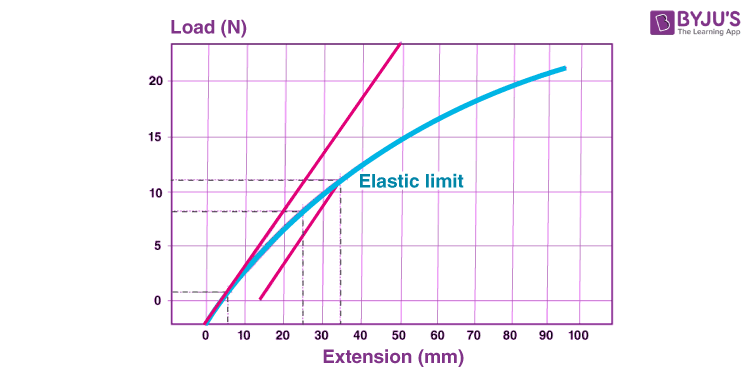# Elastic Limit

## What Is Elastic Limit?

Elastic limit is the maximum stress a material can withstand before the permanent deformation. It is the material’s highest limit before the material’s plastic deformation can occur. Once the stress or force is removed from the material, the material comes back to its original shape. Elastomers like rubber have the highest elastic limit. The behavior can be explained by Hooke’s law.

## Elastic Limit Testing

The elastic limit can be determined by measuring the greatest stress that can be applied to a given sample without causing any permanent deformation. For metals or any other rigid materials, the stress-strain curve is a straight line as the elastic limit is approximately equal to the proportional limit. Materials like rubber and plastic are called an apparent elastic limit as their stress-strain curve is not significantly straight.### What Is the Proportional Limit?

The proportional limit is defined as the highest stress at which stress and strain are directly proportional so that the stress-strain graph is a straight line such that the gradient is equal to the elastic modulus of the material. For many metals, the proportional limit is equal to the elastic limit.

### Difference between Elastic Limit and Proportional Limit

 Elastic limit Proportional limit It is defined as the point up to which the material remains elastic It is defined as the point up to which the stress and the strain are directly proportional

### Difference between Elastic Limit and Yield Point

Elastic limit and yield point are pretty much the same. But they do have a few differences as follows:

 Elastic limit Yield point Maximum stress the material can withstand before the permanent deformation It is the amount of stress present in the material before the permanent deformation

Related Physics Articles:

## What Is Hooke’s Law?## Frequently Asked Questions – FAQs

### Define Elastic limit.

Elastic limit is the maximum stress a material can withstand before the permanent deformation.

### Which type of elastomers have highest elastic limit?

Elastomers like rubber have the highest elastic limit.

### What is proportional limit?

A proportional limit is defined as the point up to which the stress and the strain are directly proportional.

### What is yield point?

The yield point is the amount of stress present in the material before the permanent deformation.

### State true or false: Natural rubber is a elastomer.

True.

To know about other Physics concepts, stay tuned with BYJU’S!

Test your knowledge on Elastic Limit